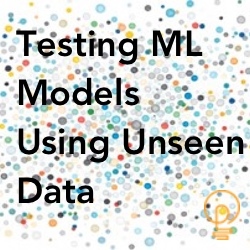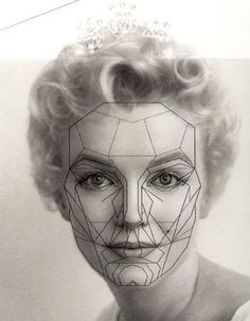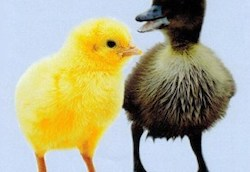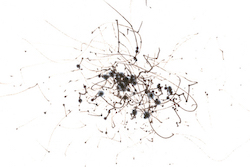## Why Our ML Models Must Be Tested With Unseen Data

Posted on 31-Mar-2020 by Craig Shallahamer / OraPub / craig@orapub.comEvaluating our machine learning models is an important step. You will sleep much better knowing you thoroughly evaluated your model before it was deployed.

However, what seems like such a simple task can become quite complicated, technical and confusing.

To understand Why "Rare Events" Can Be A Problem With Machine Learning we need to understand model accuracy and the issue with not properly testing a model. Don't worry, I will circle back to the incredibly important topic of rare events and imbalanced classification models.

In this article, I am going to explore two important concepts. First, I will introduce the simplest way to determine a model's predictive power, using a basic accuracy score. Second, I will use this score to demonstrate why we must test our trained models with data they have never seen before.

### The Beauty Of A ModelModels are not reality. They are an abstraction of reality. But models can be very useful when learning about something very complex. For example, the airflow over an wing or Oracle performance.

Most Oracle professionals are initially uncomfortable with machine learning models... but they don't know why. Perhaps it's because we feel in our gut that fancy mathematical models are not necessary to answer data related questions because a ton of data is stored in an Oracle Database.

This is kind of true and kind of false. Here's why. What if we don't have the exact data we are looking for? What if there is some missing data or we are not sure what we are looking for? For example, perhaps we have 0, 1, 2, 3, 5 and 6. But we do not have 4. The beauty of a model is it helps us understand there is a missing 4.

In machine learning, it is important to understand:

We do not develop models to memorize data.

If fact, we don't need a model to do that, we just need a little SQL with a simple WHERE clause.

We develop models to generalize and expose hidden patterns in the dataset. Then when we show the model data it's never seen before, it may be able to make a correct prediction.

### Is It A Duck Or Not A DuckFor example, supposed I wanted to teach you to recognize a duck. Your test will be to look at a picture and then tell me if the picture is a duck or not a duck.

So, I gathered ten animal pictures; 4 ducks, 3 squirrels and 3 snakes.

First, I would need to teach you what a duck looks like. So, I would show you the duck pictures, telling you that this is a duck. I would also show you the squirrel and snake pictures, telling you they are not ducks. I would repeatedly work with you until you recognized all the pictures. I would check your training by showing each picture and ask you if it is a duck or not.

Once you were able to do this perfectly, I would say you have been trained! Why? Because you correctly identified all ten pictures as either a duck or not a duck. This means your accuracy is 100% or 10 correct predictions out of 10 pictures. Let's call this score the model "training accuracy."

Let's do something similar using Python and with a dataset of 1000 samples. The "pictures" are made up of two real numbers and stored in the X array. The duck (1) or not a duck (0) labels are stored in the y array.

If you look closely at the "make_classification" function below, you'll notice I asked for 90% 1s and 10% 0s.

``````import numpy  as np
from collections import Counter
from sklearn.datasets import make_classification
from sklearn.metrics import accuracy_score

# define dataset: 2 features(X) and 1 label (y)
X, y = make_classification(n_samples=1000, n_features=2, n_redundant=0, n_clusters_per_class=1, weights=[0.90], flip_y=0, random_state=2)

# summarize data, class distribution
print("Shapes:", X.shape, y.shape)
print(Counter(y))

# define and initialize model
from sklearn import tree
myModel = tree.DecisionTreeClassifier()

# fit model and make predictions
myModel.fit(X, y)
Ypred = myModel.predict(X)

# calculate accuracy
print("Model accuracy:", accuracy_score(y, Ypred))
``````

With your Python machine learning sandbox setup, copy and paste the above code at the command line prompt. Here are the results on my system.

``````\$ python
Python 3.7.4 (default, Aug 13 2019, 15:17:50)
[Clang 4.0.1 (tags/RELEASE_401/final)] :: Anaconda, Inc. on darwin
>>> import numpy  as np
>>> from collections import Counter
>>> from sklearn.datasets import make_classification
>>> from sklearn.metrics import accuracy_score
>>>
>>> # define dataset: 2 features(X) and 1 label (y)
... X, y = make_classification(n_samples=1000, n_features=2, n_redundant=0, n_clusters_per_class=1, weights=[0.90], flip_y=0, random_state=2)
>>>
>>> # summarize data, class distribution
... print("Shapes:", X.shape, y.shape)
Shapes: (1000, 2) (1000,)
>>> print(Counter(y))
Counter({0: 901, 1: 99})
>>>
>>> # define and initialize model
... from sklearn import tree
>>> myModel = tree.DecisionTreeClassifier()
>>>
>>> # fit model and make predictions
... myModel.fit(X, y)
DecisionTreeClassifier(ccp_alpha=0.0, class_weight=None, criterion='gini',
max_depth=None, max_features=None, max_leaf_nodes=None,
min_impurity_decrease=0.0, min_impurity_split=None,
min_samples_leaf=1, min_samples_split=2,
min_weight_fraction_leaf=0.0, presort='deprecated',
random_state=None, splitter='best')
>>> Ypred = myModel.predict(X)
>>>
>>> # calculate accuracy
... print("Model accuracy:", accuracy_score(y, Ypred))
Model accuracy: 1.0
``````

Notice the final "Model accuracy" is 1.0. This means 100% of the predictions where correct. That is fantastic. You think so? Maybe. Maybe not!

### Testing Your Model With Unseen DataAn accuracy of 100% is fantastic. But perhaps you simply memorized the duck pictures and the machine learning model memorized all 1000 of its "pictures."

A good test would be to show you pictures that you have never seen before! So, I set out to find a different set of 10 pictures.

I show you the first picture and you shout, "I've never seen that picture before!" My response would be, "I did not ask you if you have seen this exact picture before. I am asking you if this is a picture of a duck." Did you notice the difference?

Being human, you would think about the attributes of a duck, such as two webbed feet, long neck, small head and a beak. If you can do that, then you will be very good at determining if a picture is a duck or not a duck.

Suppose my new set of pictures contains 5 ducks, 2 chipmunks, 1 squirrel, 1 swan and 1 flamingo. If you correctly recognized each picture as a duck or not duck, then your "testing accuracy" would be 100%, that is, 10 correct predictions out of 10 pictures.

But suppose you said the swan and the flamingo are ducks. This means your testing accuracy was 80%, that is 8 correct predictions out of 10 pictures.

Let's jump back to my machine learning example with a "test" dataset of 250 members. Just as with our training data, the "pictures" are made up of two real numbers and stored in the X array. The duck (1) or not a duck (0) labels are stored in the y array.

If you look closely at the "make_classification" function below, notice I still asked for 90% 1s and 10% 0s, but only 250 samples. I also used a different random number seed to reduce the chances of the testing data matching the training data.

It's critical to notice below, that I did not re-fit (i.e., re-train) the model. I am using the existing model that was created and trained above using the 1000 member training data. The variable name of this model is, myModel.

``````# define testing dataset: 2 features(X2) and 1 label (y2)
X2, y2 = make_classification(n_samples=250, n_features=2, n_redundant=0, n_clusters_per_class=1, weights=[0.90], flip_y=0, random_state=88)

# summarize data, class distribution
print("Shapes:", X.shape, y.shape)
print(Counter(y))

Y2pred = myModel.predict(X2)

# calculate accuracy
print("Model accuracy:", accuracy_score(y2, Y2pred))
``````

If you ran the above code, you will see this:

``````>>> # define testing dataset: 2 features(X2) and 1 label (y2)
... X2, y2 = make_classification(n_samples=250, n_features=2, n_redundant=0, n_clusters_per_class=1, weights=[0.90], flip_y=0, random_state=88)
>>>
>>> # summarize data, class distribution
... print("Shapes:", X.shape, y.shape)
Shapes: (1000, 2) (1000,)
>>> print(Counter(y))
Counter({0: 901, 1: 99})
>>>
>>> Y2pred = myModel.predict(X2)
>>>
>>> # calculate accuracy
... print("Model accuracy:", accuracy_score(y2, Y2pred))
Model accuracy: 0.888
``````

The calculated model accuracy is 0.888. This means 89% of the predictions where correct. That is pretty good. Perhaps.

You may be wondering why the model accuracy score was not 100%. After all our model was training with 1000 "pictures." Apparently, our model is fantastic at memorizing data (100%) and pretty good at recognize similar patterns (89%)... but not perfect.

The larger the gap between training accuracy and testing accuracy, the more a model has simply memorized data. Our desire is for both a high and similar accuracy score.

In fact, I woud much rather have a train and test accuracy of 87% then a training accuracy of 97% and a testing accuracy of 87%.

### A Better Way To Train And TestTraining and testing our data is so central to developing a proper machine learning model, there are functions already written for us to help make this easier. These pre-built functions also do some advanced stuff that make our models even better.

But that's a topic for another article.

All the best in your machine learning work,

Craig.

Start my FREE 18 lesson Machine Learning For Oracle Professionals E-Course here.Craig Shallahamer is a long time Oracle DBA who specializes in predictive analytics, machine learning and Oracle performance tuning. Craig is a performance researcher and blogger, consultant, author of two books, an enthusiastic conference speaker a passionate teacher and an Oracle ACE Director. More about Craig Shallahamer...

If you have any questions or comments, feel free to email me directly at craig at orapub.com.